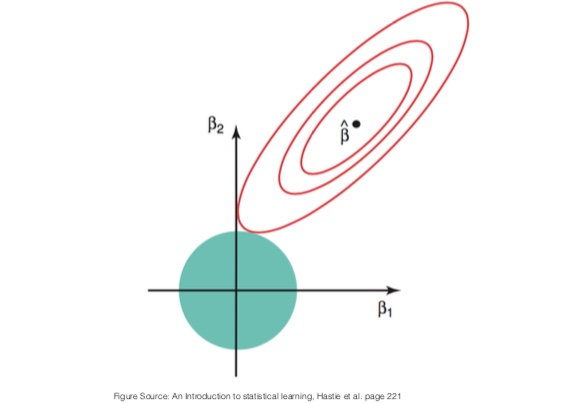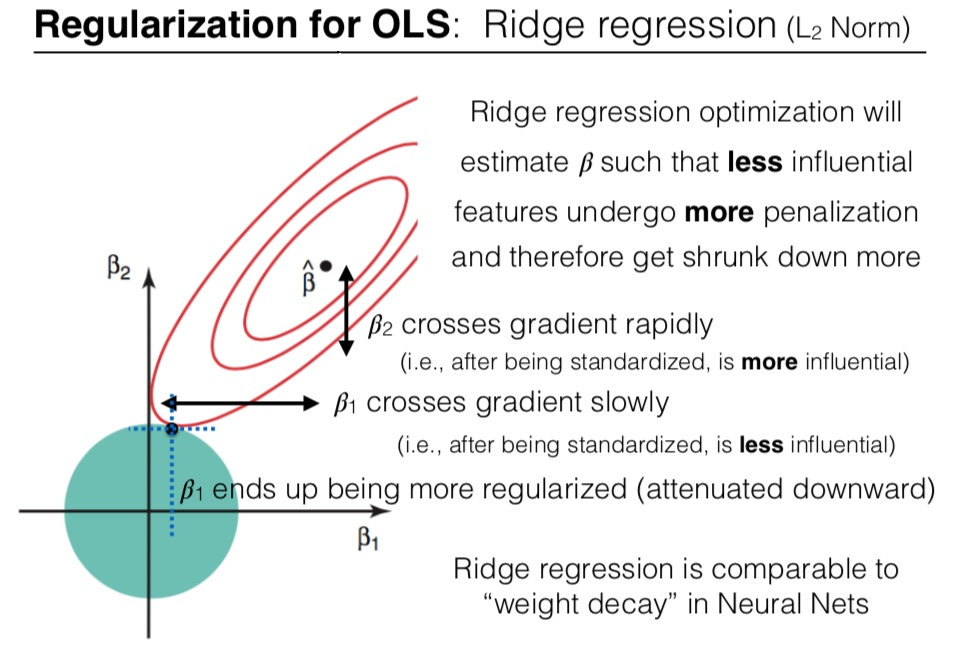Machine-learning 5 minutes

# The effect of L2-regularization

In this article, we discuss the impact of L2-regularization on the estimated parameters $\vw$ of a linear model.

## What is L2-regularization

L2-regularization adds a regularization term to the loss function. The goal is to prevent overfiting by penalizing large parameters in favor of smaller parameters. Let $\sets$ be some dataset and $\vw$ the vector of parameters:

Where $\lambda$ is an hyperparameter that controls how important the regularization

## The effect of the hyperparameter

Increasing the $\lambda$ hyperparameter moves the optimal of $\l_\text{reg}$ closer to $0$, and away from the optimal for the unregularized loss $\l$.

This can be visualized using one feature $\sx$ and a dataset made of one sample $(\sx, \sy)$. Take (for instance) the following loss:

The regularization term is:

On the graph below, we plotted this loss function (first graph) and several variant of the corresponding $\l_\text{reg}$ for different values of $\lambda = 0, 1, 2, 3, 4$. As the value of $\lambda$ increases, the loss curve is translated towards $0$.This can be vizualized in 2D, where we see that the optimum of the regularized loss approaches $\vec{0}$ more and more when $\lambda$ increases. However, there is another way to conceptualize the regularization, which we will present next.

## Regularization seen as constrained optimization

To understand how L2-regularization impacts the parameters, we will use an example in $\realvset{2}$.

Let’s note $\beta = (\beta_1, \beta_2)$ the vector of parameters.

Our estimate is:

Which is equivalent to a constrained optimization problem:

 minimize: $\l(\sets, \beta)$ subject to: $\normtwo{\beta}^2 \leq \ss^2$

This formulation is easier to interpret: the selected vector of parameters $\hat{\beta}$ is the vector that minimzes the loss, among all vector inside the ball of radius $\ss$.

This is illustrated on the picture below. The red contour lines are the contour lines of the loss function $\l$. The unregularized optimal $\hat{\beta}$ is indicated by a black dot at the location of the minimum of $\l$. The ball of radius $\ss$ is drawn in blue. The solution to the constrained optimization is the intersection of the contour lines and the ball.## Effect on the individual parameters

What is the effect of the regularization on the individual parameters $\beta_1$ and $\beta_2$ ?

Regularized optimization will estimate $\beta$ such that less influential features undergo more penalization and therefore get shrunk down more.

On the plot above, $\beta_2$ crosses the gradient more rapidly that $\beta_1$ (we can see this as the contour lines are less separated along the $\beta_2$ axis than along the $\beta_1$ axis). When both $\beta_1$ and $\beta_2$ are standardized, this means that $\beta_2$ is more influential than $\beta_1$.

As a result, $\beta_1$ is more penalized by the L2-constraint than $\beta_2$.This phenomenon explains why we should normalize the features before using regularization. More details on this in what follows.

## Effect when approaching 0

Since the gradient of $\normtwo{\cdot}$ vanishes around $0$, the optimal will never move there if it was not already there. In other words: the parameters $\beta_\si$ are pushed towards $0$, but they are never set to $0$.

Another regularization method, the L1 regularization has a different behavior: since the gradient around $0$ do not vanish, the parameters $\beta_\si$ are pushed towards $0$ and may attain it and remain there. is.

## Important remark about normalization

The regularizer term $\normtwo{\vw}^2$ treats each component the same way:

Therefore it is important to unify the scale of each feature vector $\vf_\si$. Indeed, suppose that the optimal solution is $\hat{\vw}$ for some design matrix $\mx$ where:

The estimate $\hat{\vy}$ is:

If we rescale one column of the design matrix, say $\vf^{r}_{0} = \epsilon\vf_{0}$, then we don’t bring new information and the estimate $\hat{\vy}$ should not change. The parameter $\sw^{r}_{0}$ is inversely rescaled:

And:

But the parameters $\sw_0$ and $\sw^{r}_0 = \epsilon^{-1}\sw_0$ will be penalized with the same strength by the L2 regularization.

Therefore, to avoid mistaking influential parameters with non-influential ones because of scaling factors within the feature vector, we must normalize the features.

By norming the columns of $\mx$, we put them on the same scale. Consequently, differences in the magnitudes of the components of $\vw$ are directly related to the wiggliness of the regression function $\mx\vw$, which is loosely speaking what the regularization tries to control.

TL;DR: before using regularization, transform your feature vectors into unit vectors.